# Attention机制变体的介绍与对比

## hard attention vs soft attention

《Recurrent Models of Visual Attention》中Volodymyr Mnih提出了hard attention方法。

《Neural Machine Translation by Jointly Learning to Align and Translate》中Dzmitry Bahdanau提出了soft attention的方法。

《Show, Attend and Tell: Neural Image Caption Generation with Visual Attention》中Kelvin Xu将这两种方法在Image Caption进行了比较，两种方案生成的注意力效果如下图所示。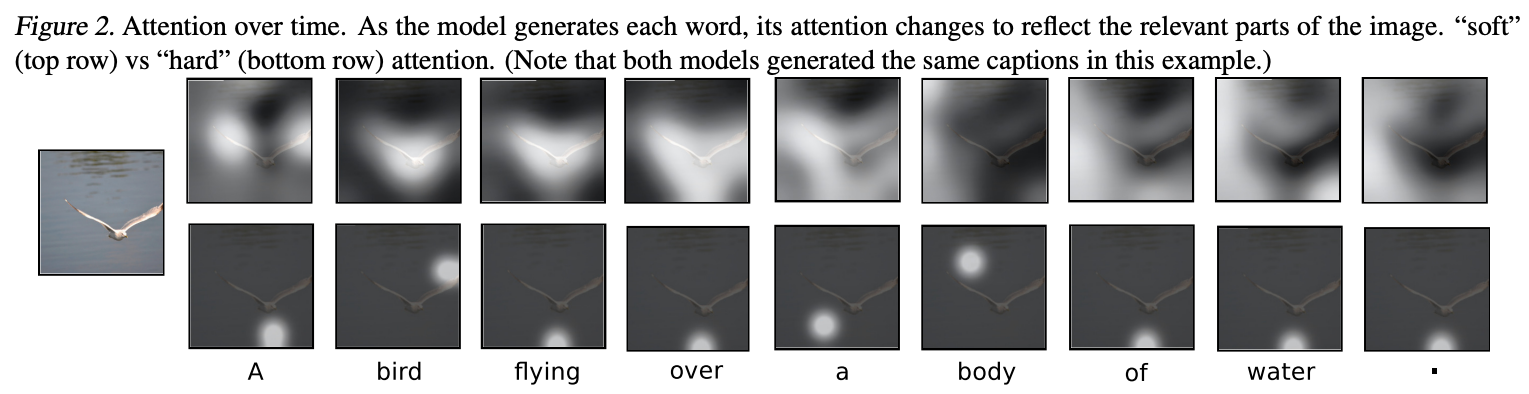### soft attention

• 平均的方法得到Z，对所有区域都关注，只是关注的重要程度不一样。
• 整个模型都是平滑的，可微分的，可以用标准的反向传播算法进行学习。
• 是一种确定（deterministic）的学习过程。

soft attention计算过程
x1~Xn分别覆盖图像的一个子部分。为了计算分数 si 来衡量对 xi 的关注程度，我们使用（上下文 C=ht−1）：

$s_{i}=\tanh \left(W_{c} C+W_{x} X_{i}\right)=\tanh \left(W_{c} h_{t-1}+W_{x} x_{i}\right)$

$\alpha_{i}=\operatorname{softmax}\left(s_{1}, s_{2}, \ldots, s_{i}, \ldots\right)$

$Z=\sum_{i} \alpha_{i} x_{i}$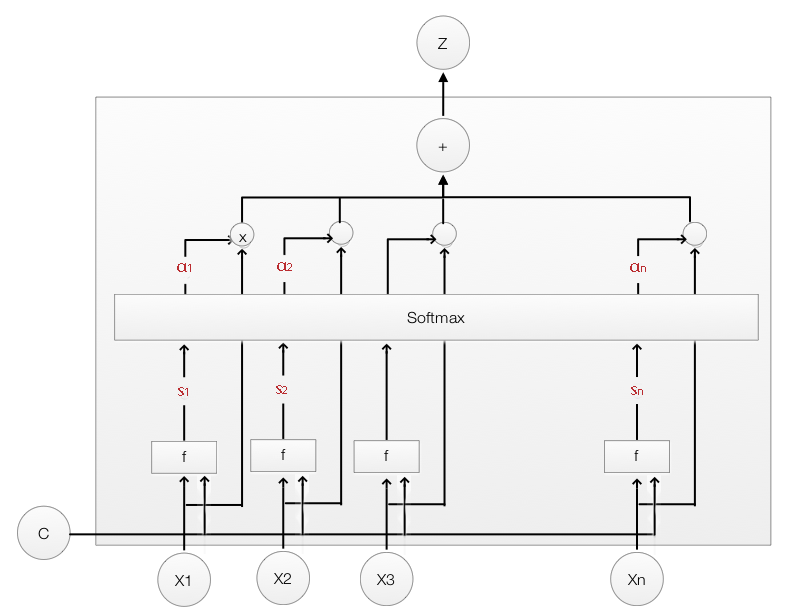soft attention的注意力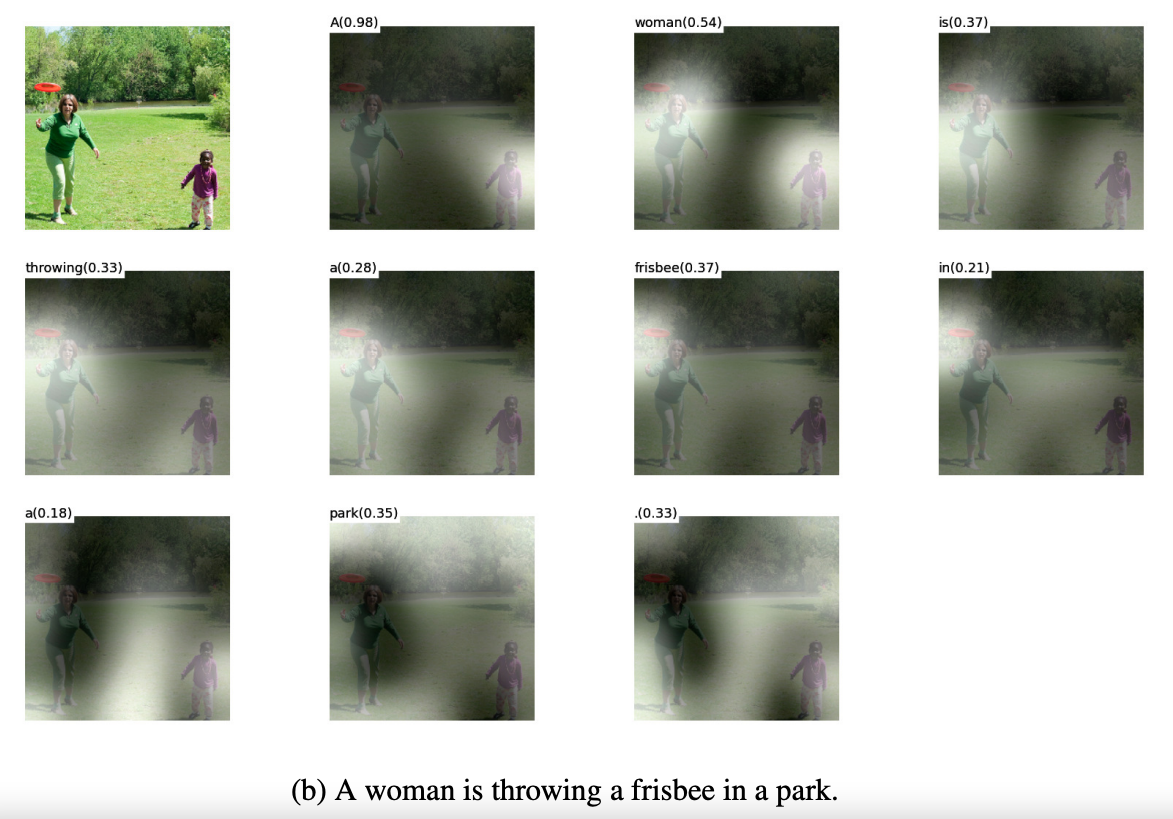### hard attention

• 采样得到Z，权重服从贝努利分布，非0即1，对特定时间特定区域只有关注与不关注。
• 不连续不可导，无法在反向传播中利用梯度更新，使用类似reinforcement learning的方法进行学习。
• 是一种随机（stochastic）的学习过程。

hard attention计算过程
x1~xn分别覆盖图像的一个子部分。我们为每个xi计算一个权重αi，并使用它来计算xi作为LSTM输入的加权平均值。αi加起来为1，可以解释为xi是我们应该关注的区域的概率。因此，hard attention不是加权平均值，而是使用αi作为采样率来选择一个xi作为 LSTM 的输入。

$Z \sim x_{i}, \alpha_{i}$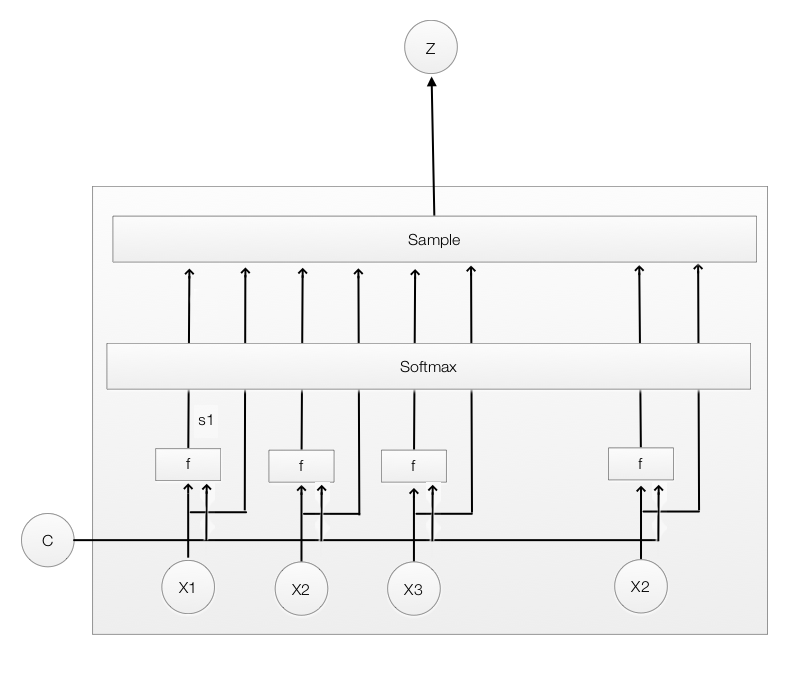hard attention的注意力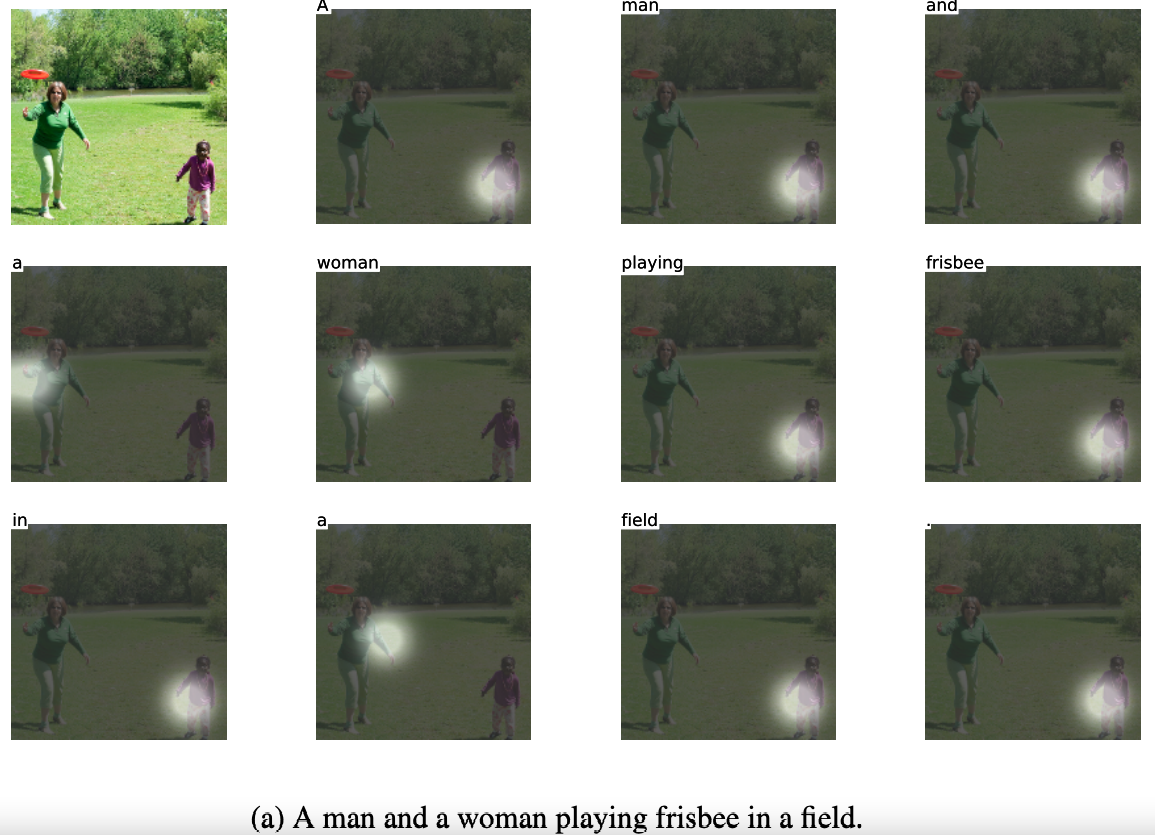## global attention vs local attention

global attention和local attenion的区别在于“注意力”是放在所有源位置还是仅放在几个源位置。
《Effective Approaches to Attention-based Neural Machine Translation 》中，Luong做了详细的说明和对比。

### global attention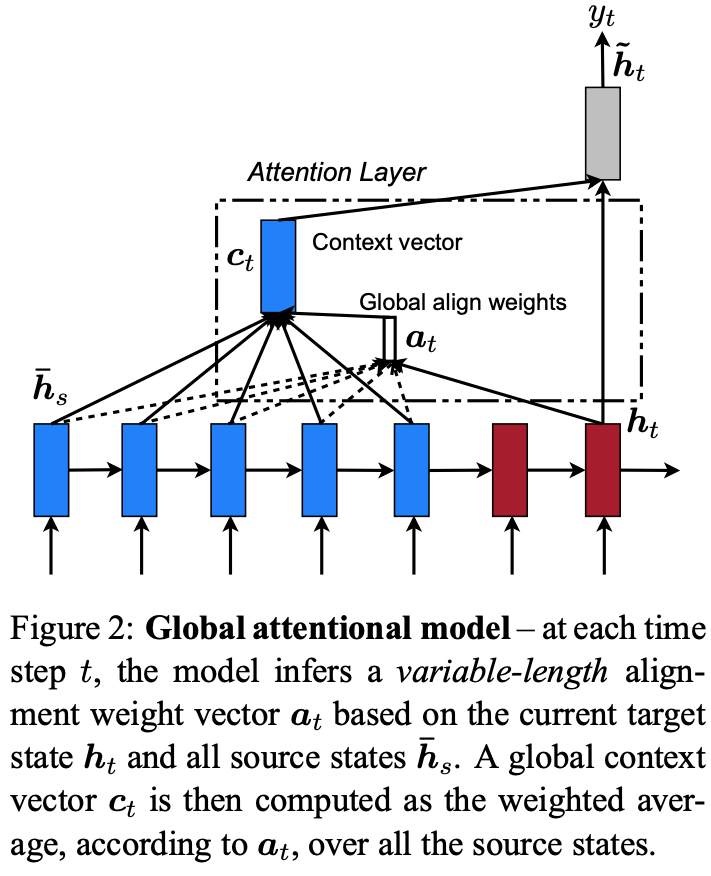\begin{aligned} \boldsymbol{a}_{t}(s) &=\operatorname{align}\left(\boldsymbol{h}_{t}, \overline{\boldsymbol{h}}_{s}\right) \\ &=\frac{\exp \left(\operatorname{score}\left(\boldsymbol{h}_{t}, \overline{\boldsymbol{h}}_{s}\right)\right)}{\sum_{s^{\prime}} \exp \left(\operatorname{score}\left(\boldsymbol{h}_{t}, \overline{\boldsymbol{h}}_{s^{\prime}}\right)\right)} \end{aligned}

$\operatorname{score}\left(\boldsymbol{h}_{t}, \overline{\boldsymbol{h}}_{s}\right)=\left\{\begin{array}{ll} \boldsymbol{h}_{t}^{\top} \overline{\boldsymbol{h}}_{s} & \text { dot } \\ \boldsymbol{h}_{t}^{\top} \boldsymbol{W}_{\boldsymbol{a}} \overline{\boldsymbol{h}}_{s} & \text { general } \\ \boldsymbol{v}_{a}^{\top} \tanh \left(\boldsymbol{W}_{\boldsymbol{a}}\left[\boldsymbol{h}_{t} ; \overline{\boldsymbol{h}}_{s}\right]\right) & \text { concat } \end{array}\right.$

### local attention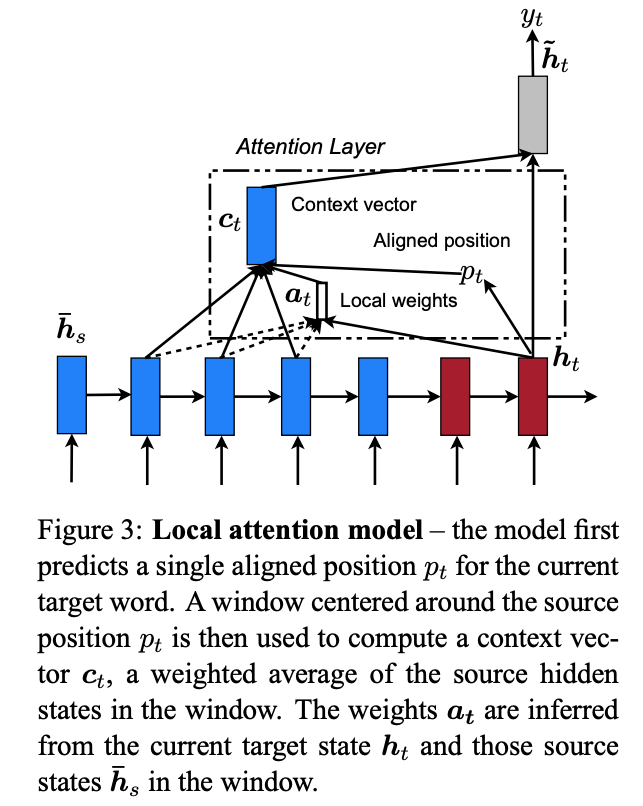monotonic alignment(local-m)

predictive alignment(local-p)

$p_{t}=S \cdot \operatorname{sigmoid}\left(\boldsymbol{v}_{p}^{\top} \tanh \left(\boldsymbol{W}_{\boldsymbol{p}} \boldsymbol{h}_{t}\right)\right)$

\boldsymbol{a}_{t}(s)=\operatorname{align}\left(\boldsymbol{h}_{t}, \overline{\boldsymbol{h}}_{s}\right) \exp \left(-\frac{\left(s-p_{t}\right)^{2}}{2 \sigma^{2}}\right)

### 总结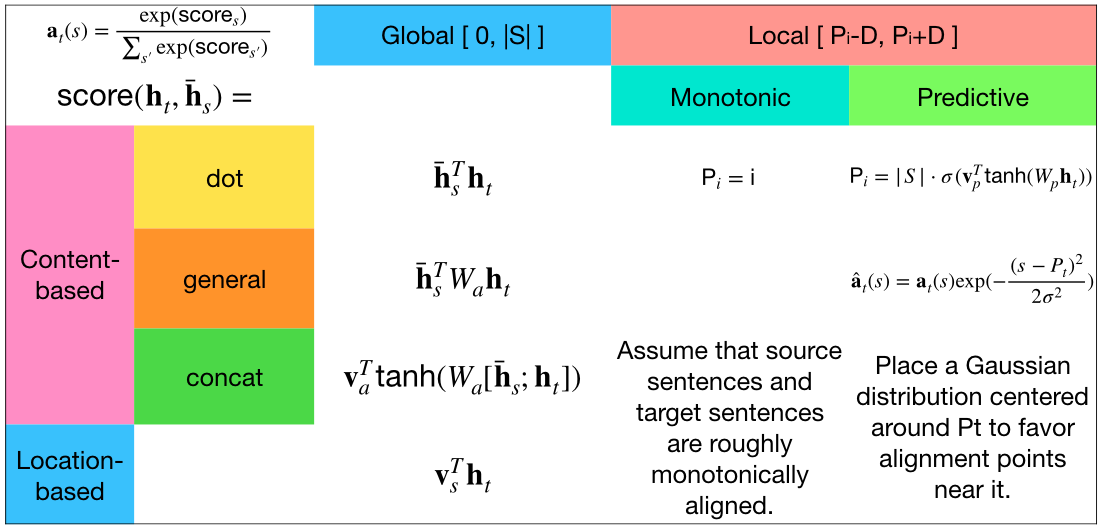## bahdanau attention vs luong attention

luong attention和bahdanau attention是比较流行和经典的两种attention机制实现，是用作者名字命名的，分别是在Minh-Thang Luong的《Effective Approaches to Attention-based Neural Machine Translation》和Dzmitry Bahdanau的《Neural Machine Translation by Jointly Learning to Align and Translate》中被提出来的方法。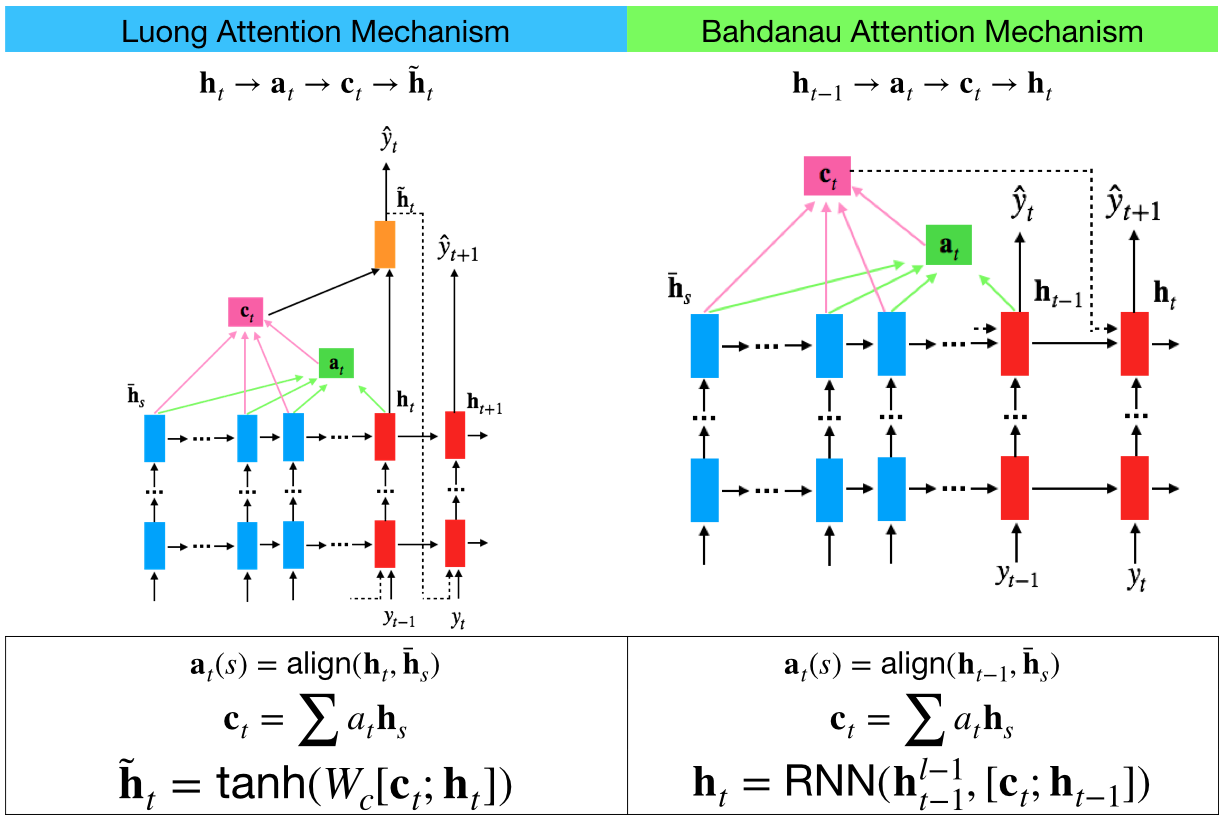1.在Bahdanau Attention机制中，第t步的注意力对齐中，使用的是Decoder中第t-1步的隐藏状态$h_{t-1}$和Encoder中所有的隐藏状态$\overline{\mathbf{h}}_{s}$加权得出的，但是在Luong使用的是第t步的隐藏状态$h_{t}$
2.在Bahdanau Attention机制中，decoder在第t步时，输入是由$c_t$和Decoder第t-1步的隐藏状态$h_{t-1}$拼接得出的，得到第t步的隐藏状态$h_{t}$并直接输出$\hat{\mathbf{y}}_{t+1}$。而 Luong Attention 机制在 decoder部分建立了一层额外的网络结构，输入是有$c_t$和Decoder第t步的隐藏状态$h_{t}$拼接作为输入，得到第t步的隐藏状态$\tilde{\mathbf{h}}_{t}$并输出$\hat{\mathbf{y}}_{t}$
3.Bahdanau Attention 机制只尝试了concat作为对齐函数，而Luong Attention 机制的论文在多种对齐函数上做了实验。